# Brian

Brian divides 80 by 7. Between what two whole numbers is his answer?

a =  11
b =  12

### Step-by-step explanation:

$b=⌈x⌉=⌈11.4286⌉=12$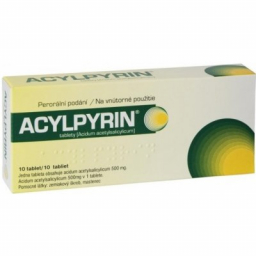Did you find an error or inaccuracy? Feel free to write us. Thank you!Tips to related online calculators
Do you want to round the number?
Do you want to perform natural numbers division - find the quotient and remainder?

## Related math problems and questions:

• EstimateEstimate the answer by rounding each fraction to the nearest whole number and then subtracting. 9/15 - 2 7/8
• Yogurt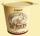The board is 35 yogurts. How many boards of yogurts must be ordered for 886 people in the canteen?
• RemainderWhat is the remainder of the division of natural numbers 293 and 7?
• Closest natural numberFind the closest natural number to 4.456 to 44.56 and 445.6.
• Division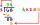Divide by the number 0.2 is the same as multiply by what number?
• FortyForty five of the 80 students were girls. What is the ratio of girls to boys?
• NumbersDetermine the number of all positive integers less than 4183444 if each is divisible by 29, 7, 17. What is its sum?
• DivisibilityDetermine all divisors of number 84.
• Find unknown numberWhat is the number between 50 and 55 that is divisible by 2,3,6,9?
• How manyHow many integers are greater than 547/3 and less than 931/4?
• Evaluate 12Evaluate and simplify 9 divided by 7/6.
• Two numbers 6Fill two natural numbers a, b: 7 + blank- blank = 5
• Whole numbersPavol wrote down a number that is both rational and a whole number. What is one possible number she could have written down?
• Reciprocal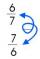Calculate the reciprocal numbers for the given real numbers.
• Sum and roundingI know two numbers whose sum is 20. When they each round and then added together I get the 30. What are this numbers?
• Divisible by fourAre 240 divisible by 4?
• Twelve eggs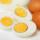A dozen eggs cost 80 cents . how much will 3½ dozen eggs cost?